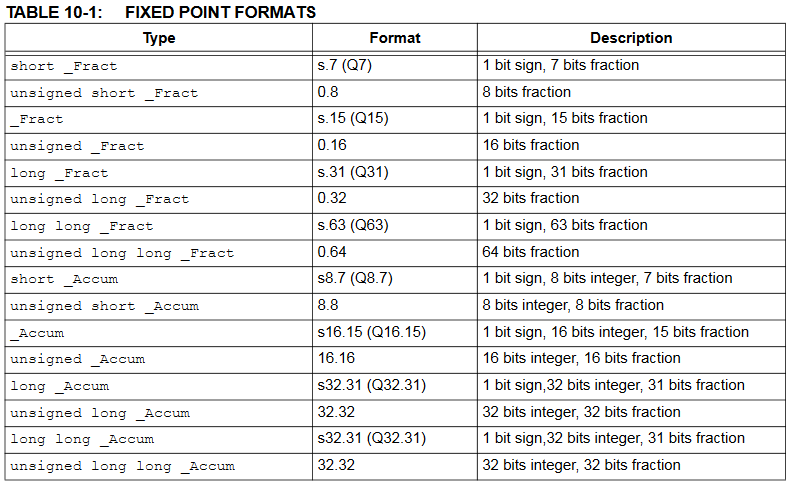Cornell University ECE4760
Fixed Point Arithmetic

PIC32MX250F128B

Introduction

Fixed point arithemetic is generally faster than floating point on the PIC32MX, and often has enough dynamic range and accuracy for animation and DSP. The version of the compiler we use natively supports fixed point. See XC32 Compiler Users Guide, Chapter 10. The types are given below in a table from the Users Guide. In the table, the s represents a sign bit. The type _Accum is a signed 16-bit integer, 15-bit fraction format with a range of +/-65536 and a resolution of 3x10-5.
To access the fixed point types, use: #include <stdfix.h>

Before the native support, I decided to implement s2.30, s16.16 (similar to _Accum) and s2.14 formats. The format s2.30 means two bits to the left of the binary-point, one of which is the sign bit. Fixed point is used in DSP, animation loops, and control loops where speed is the limiting factor. There is a table below comparing perfromance of my fixed point and the native fixed point.Compiler Support for fixed-point types in this table includes:
• prefix and postfix increment and decrement operators ( ++ , -- )
• unary arithmetic operators ( + , - , ! )
• binary arithmetic operators ( + , - , * , / )
• binary shift operators ( << , >> )
• relational operators ( < , <= , >= , > )
• equality operators ( == , != )
• assignment operators ( += , -= , *= , /= , <<= , >>= )
• conversions (casts) to and from integer, floating-point, or fixed-point type
• Defining literal fixed point constants. See section 10.3 of XC32 Compiler Users Guide
NOT SUPPORTED: printf or scanf conversion of fixed types. Workaround: cast to/from float or int, as appropriate.

An example code defines numbers using my fix16, the GCC standard _Accum, and GCC 32-bit float and compares performance for add, multiply, and divide.
The cycles/operation includes getting the operands from memory and storing the result.
Cycle count comes from reading timer 2.

Cycles/operation fix16 _Accum float
Multiply 21 28 ~55
Divide ~145 ~145 ~140
DSP-MAC 21 29 ~110

Another code defines numbers using my fix16, and fix14 , and the GCC standards _Accum, and _Fract and compares performance for add, multiply, and MAC.
The cycles/operation includes getting the operands from memory and storing the result.

Cycles/operation fix16 _Accum fix14 _Fract
Multiply 19 27 9 21
DSP-MAC 18 28 10 24

My Fixed point arithmetic systems (but you should use the native types)

--16.16-- fix16 format
For animation, another fixed point system useful over a larger integer range is 16.16 format with a range of +/-32767 and a resolution of 1.5x10-5. This is the system used in the particle animations. The TFT_animation_BRL4.c example on the ProtoThreads page uses 16.16 to simulate a projectile.
The macros for this system are:

`typedef signed int fix16 ;#define multfix16(a,b) ((fix16)(((( signed long long)(a))*(( signed long long)(b)))>>16)) #define float2fix16(a) ((fix16)((a)*65536.0)) // 2^16#define fix2float16(a) ((float)(a)/65536.0)#define fix2int16(a)    ((int)((a)>>16))#define int2fix16(a)    ((fix16)((a)<<16))#define divfix16(a,b) ((fix16)((((signed long long)(a)<<16)/(b)))) #define sqrtfix16(a) (float2fix16(sqrt(fix2float16(a)))) #define absfix16(a) abs(a)`

--2.30--
The dynamic range of the 2.30 is -2 to 2-2-30. The resolution is 2-30=9*10-10. The high resolution is necessary to make stable, very accurate, IIR filters. The dynamic range is sufficient for Butterworth, IIR filters, made with second order sections (SOS). SOS help to minimize filter roundoff errors. The macros for the 2.30 follow:

```typedef signed int fix32 ;
#define multfix32(a,b) ((fix32)(((( signed long long)(a))*(( signed long long)(b)))>>30)) //multiply two fixed 2:30
#define float2fix32(a) ((fix32)((a)*1073741824.0)) // 2^30
#define fix2float32(a) ((float)(a)/1073741824.0)
```

--2.14--
A narrower 2.14 format is good for fast, reasonable accuracy filters. This is 2-bit integer (sign bit and one bit) with 14-bit fraction (2.14 format). Range is -2 to 2-2-14 and resolution is 2-14=6*10-5.
For more poles than 2-pole IIR, you must use Second-Order-Section filters (example 6 on DSP page).The macros for this system are:
// == bit fixed point 2.14 format ===============================
// == resolution 2^-14 = 6.1035e-5
// == dynamic range is +1.9999/-2.0
typedef signed short fix14 ;
#define multfix14(a,b) ((fix14)((((long)(a))*((long)(b)))>>14)) //multiply two fixed 2.14
#define float2fix14(a) ((fix14)((a)*16384.0)) // 2^14
#define fix2float14(a) ((float)(a)/16384.0)
#define absfix14(a) abs(a)

Note that there are no integer conversion macros for this format because the integer range is only +/-2.

Fixed point arithmetic performance

The performance for operations vary, but fixed point is faster than floating point on this architecture. At optimization level -O1, the following table gives the timing in cpu cycles for a multiply operation and a multiply-accumulate (MAC) operation. MAC is common in DSP. Also given is the number of assembler opcodes executed (To see assembler listing in MPLABX use menu item Window>Debugging>output>Dissasembly).
The times in the table include the time to load/store variables. For 16.16 operations some of the multiplies are 2 cycles. (Code)

multiply
cycles

multiply
opcodes
MAC
cycles
MAC
opcodes
fix14 8 8 9 9
fix16 12 10 20 16
float 50-53 library call 99-119 library call

Fixed multiply for 16.16 is about 4 times faster than floating point, and 2.14 multiply is about 6.5 times faster than floating point. Other operations do not have as great a ratio. For example, fixed 16:16 divide is the same speed as float, and fixed square root is 0.6 the speed of the float operation. Fortunately, DSP uses only add and multiply, in about equal numbers. Compiling without optimization does not slow down floating point (probably because the libraries are optimized), but slows the fixed operations about a factor of 2-3.

Copyright Cornell University July 2, 2019